Family of Circles for IIT JEE

Types of Circles - Concentric circles, Contact of circles, Orthogonal circles

Introduction of family of circle

A circle is a closed shape simple figure in which all the points lying on the surface of the circle joining its centre are equal and known as radius of the circle. A huge collection of circles is called a family of circles. There are various types of circles available around us. Family of circles is one of the most important parts of coordinate geometry in which different circles are present at the same time, through which the calculations to find the values in circles becomes easy. The circles consisting of the same properties are combined together in various ways to form the family of circles. The general equation of the circle can be given as x2 + y2 + 2gx + 2fy + c = 0. However, the equation contains three unknown figures i.e. g, f and c so we require at least three conditions to get a unique circle.

For instance, if we are given two circles and we need to resolve the third circle touching the rest both the circles. For this, we need one more condition. Without the condition we get the equation of family of circles which satisfies the given two conditions. Imposition of a third condition will result in the equation which represents a particular circle.

Let’s discuss some of the ways of finding the family of the circle when you are given with certain conditions:

• 1. When family of circles have a fixed centre

• The equation is (x-y) 2 + (y-k) 2 = r2
Where (h, k) is fixed and the only parameter that is varying is r. The fixation of the radius will give a particular circle.

• 2. Equation of family of circles passing through intersection of two circles S1 = 0 and S2 = 0.

• The general equation of family of circles is passing through the intersection of S1 and S2 which is given by S1 + KS2 = 0, where k ≠ -1. Again we are left with one parameter (k) equation of the family of circles. Further, the parameter value of the parameter gives a unique circle.

Caution:
If k = –1, we will get equation of common chord i.e. straight line instead of circle.  Let S1 ≡ x2 + y2 + 2g1x + 2f1y + c1 = 0
S2 ≡ x2 + y2 + 2g2x + 2f2y + c2 = 0
Since, point lies on both the circles,
⇒ xA2 + yA2 + 2g1xA + 2f1yA + c1 = 0
So, xA2 + yA2 + 2g2xA + 2f2yA + c2 = 0
⇒ xA2 + yA2 + 2g1xA + 2f1yA + c1 + λ (xA2 + yA2 + 2g1xA + 2f1yA + c1) = 0
⇒ Point A (xA, yA) lies on S1 + λ S2 = 0 ∀ λ ∈ R
Similarly point B (xB, yB) lies on S1 + λ S2 = 0 ∀ λ ∈ R
Therefore, S1 + λ S2 = 0 is the family of circles through the intersection of S1 = 0 and S2 = 0.

• 3. Equation of the circle circumscribing a triangle whose sides are given by L1 = 0, L2 = 0 and L3 = 0.

• This equation is given by L1L2 λL2L+ µLL= 0, that provide coefficient of x y= 0 and coefficient of x2 = coefficient of y2. Moreover, the particular value of the parameter λ and µ gives you a unique circle.

• 4. Family of circle touching the circle S= 0 and the line L = 0 at their point of contact

• The family is given by equation S + λL = 0, where λ is a required family.

• 5. Family of circle passing through two mention points i.e. A(x1, x1) and B(x2, y2).

• The equation of the circle is (x – x1) (x – x2) + (y – y1) (y – y2) = 0 having AB is its diameter and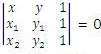is the equation of a line passing through the points A and B.

Required equation of family of circles is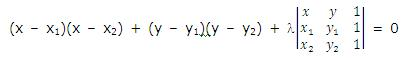• 6. Family of circles touching the given line L = 0 at a point (x1, y1) on the line is

• x – x1)2 + (y – y1)2 + λL = 0 is the particular value of the parameters λ which gives a unique circle. The equation of the family of circles touches the line y – y1 = m (x – x1) at (x1, y1) for any values of m is (x – x1)2 + (y – y1)2 + λ[(y – y1) – m(x – x1)] = 0.
Here, we can have two sub-cases according as whether the line is parallel to x -axis or y-axis.

If this line through (x1, y1) is parallel to x-axis, the equation of the family of circles touching it at (x1, y1) becomes (x – x1)2 + (y – y1)2 + K(y – y1) = 0.

Moreover, if the line through (x1, y1) is parallel to x-axis, then the equation of the family of circles touching it at (x1, y1) becomes (x – x1)2 + (y – y1)2 + K(y – y1) = 0.

• 7. Equation of circle circumscribing a quadrilateral whose sides in order are expressed by the lines L1 = 0, L2 =0, L3 = 0 and L4 = 0.

• The equation of the required family is given by L1L+ λL2L= 0 which provide coefficient of x2 = coefficient of y2 and coefficient of xy = 0.

Types of circles

• 1. Concentric circles

• When two or more circles have the common centre, then these circles are called concentric circles.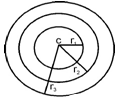In the above figure, there are three circles inside one other. All these circles are of different size and are having different radius. All circles have one common centre i.e. C but not the common radius. Like in this given figure, the first inner circle is having radius1, middle circle is having radius2 and third outer circle is having radius3. It means that all the three circles have different measurement having the same centre. Consequently, if all the circles have the radius then the circles will not be concentric. As a result they will lie on each other and will not be able to see and treat each other as one single circle.
Here is the equation of concentric circle – x2 + y2 + 2gx + 2fy + c = 0 is x2 + y2 + 2gx + 2fy + k = 0 (Equation differ only by the constant term).

• 2. Contact of circles

• When outer surface of two circles are touching, it is known as contact of circles.

There may be two cases in contact of circles.

Case (i) when two circles touching the outer surface externally and where the distance between their centres is equal to the sum of their radii. In this case, circles must satisfy the given equation.

Equation – c1c2 = r1 + r2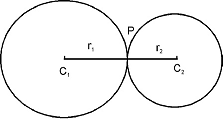In the above figure, there are two circles touching each other externally at point P. Both circles have different centres c1 and c2. The distance between both centres is the sum of their radii.

Case (i) when two circles touching the outer surface internally having their centres is equal to the difference of their radii.
Equation – c1c2 = r1 – r2 or r2 - r1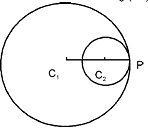In the above figure, outer of the two circles are touching internally at P point. Similarly in case (i), both circles have different centres c1 and c2

• 3. Orthogonal circles

• When two circles cut each other at right angles, they are called orthogonal circles. According to the Pythagoras theorem, two circles of radii r1 and r2 whose center area distance d apart are orthogonal, if r12 + r22 = d2. Figure as an example is given below.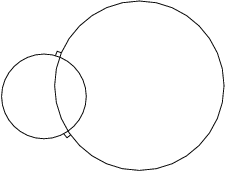We are mentioning solved example below to find the equation of a circle through the points of intersection of two given circles.

Example: Find the equation of the circle through the intersection of the circles x22 + y22 - 8x - 2y + 7 = 0 and x22 + y22 - 4x + 10y + 8 = 0 and has been passed through the point (-1, -2).

Solution - The equation of any circles passing through the intersection of the circles S11= x22 + y22 - 8x - 2y + 7 = 0 and S22 = x22 + y22 - 4x + 10y + 8 = 0 is S11 + λS22 = 0

Therefore, the equation of the required circle is (x22 + y22 - 8x - 2y + 7) + λ(x22 + y22 - 4x + 10y + 8) = 0, where λ (≠ -1) in an arbitrary real number

The point (-1, -2) has been passed through the circle. Therefore,   (1 + λ) + 4(1 + λ) + 4(2 + λ) + 4(1 - 5λ) + 7 + 8λ = 0
⇒ 24 - 3λ = 0
⇒ λ = 8
Now putting the value of λ = 8 in the equation (x22 + y22 - 8x - 2y + 7) + λ(x22 + y22 - 4x + 10y + 8) = 0, we get the required equation as 9x22 + 9y22 – 40x + 78y + 71 = 0.